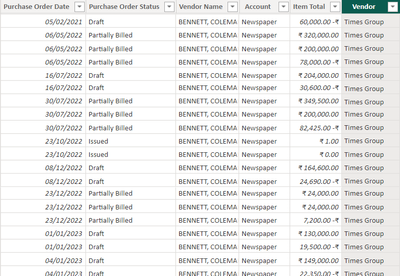cancel
Showing results for
Did you mean:Regular Visitor

## Need to calculate total incentive from all the vendors based on the deal period and incentive %

Hi,

I have a transaction table "Zoho Dump" with the purchase order date and item total and I need to calculate the incentive as per the incentive slab in the Incentives table.Total Sales = SUM('Zoho Dump'[Item Total])

Sales in deal period = CALCULATE([Total Sales],DATESBETWEEN('Date'[Date],MAX(Vendors[Deal Start]),MAX(Vendors[Deal End])))

Sales Slab = CALCULATE(ALLSELECTED(Incentives[Slabs]),FILTER(Incentives,[Sales in deal period]>Incentives[Slab Start]&&[Sales in deal period]<=Incentives[Slab End]))

Incentive % = CALCULATE(ALLSELECTED(Incentives[Incentive %]),FILTER(Incentives,[Sales in deal period]>Incentives[Slab Start]&&[Sales in deal period]<=Incentives[Slab End]))

Incentive = [Sales in deal period]*[Incentive %]

I have created these measures for calculating the Sales in deal period, current Sales Slab as per sales, Applicable Incentive % and total incentive.

Now the issue is that there are 3 vendors for those incentive will not be calculated in this way. Incentive for those vendors will be calculated on incremental basis. Means if the slab start from 50000 and the sales is 60000 then the incentive will be applicable for 10000 not the complete 60000.

How can i exclude these 3 vendors from this calculation Incentive = [Sales in deal period]*[Incentive %]

And how can I calculate the incentive for these 3 vendors on incremental basis.

0 REPLIES 0